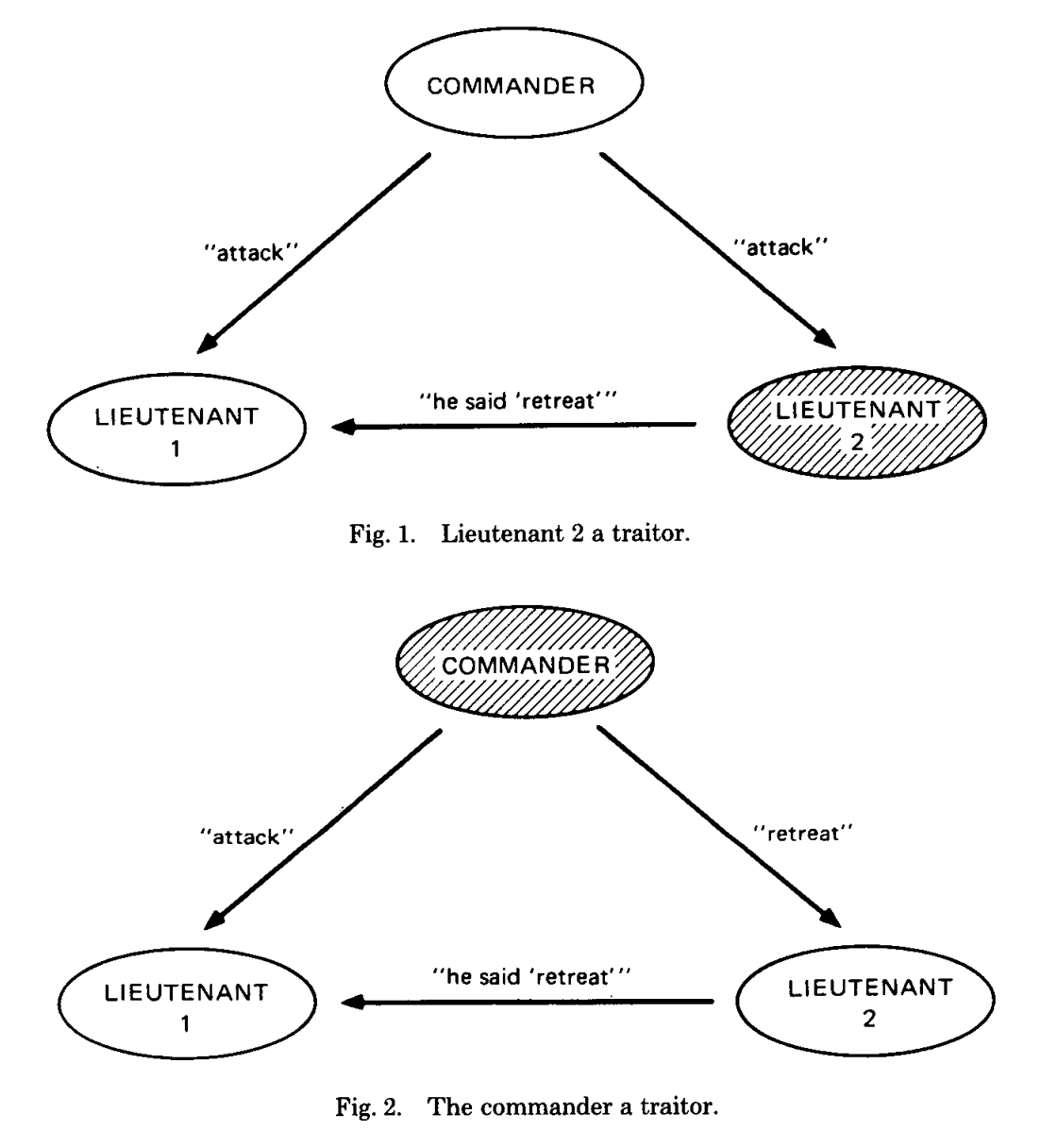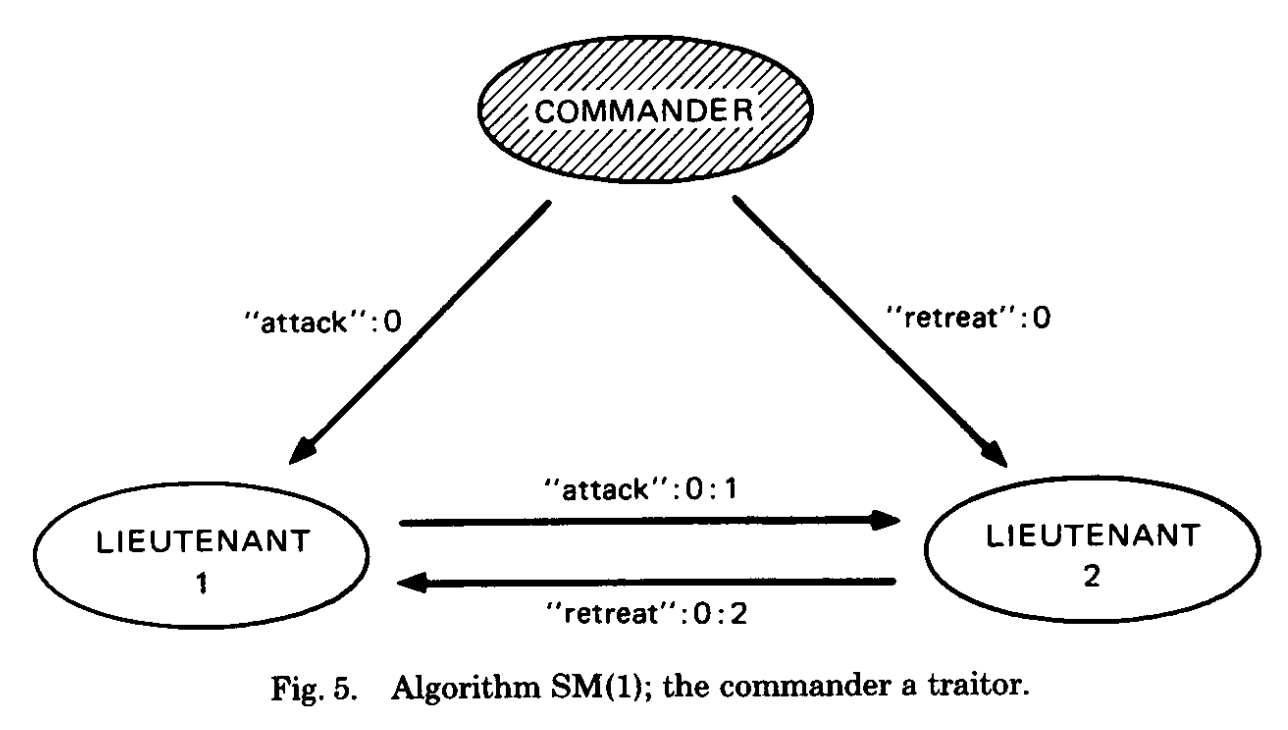fatcat 枝头不见绿，蓄势待春风

2019-11-06
fatcat22

# 什么是拜占庭将军问题

「拜占庭将军问题」来源于这样一个场景：

## 推导

A. 所有忠诚的将军可以达成一致的作战计划
B. 少数的叛徒不会导致忠诚的将军们无法达成一致

1. 所有忠诚的将军必须拿到相同的消息序列 $v(1),v(2),…,v(n)$

2. 如果将军$i$ 是忠诚的，那么它发出的原始值 $v(i)$ 必须被其他忠诚的将军所采用

1’. 对于任意一个将军$i$，无论他是忠诚的还是叛徒，当他发出值时，所有的忠诚的将军都将采用相同的值，即 $v(i)$（虽然可能不是将军$i$发送的原始值）

IC1. 所有忠诚的副官获得相同的命令
IC2. 如果司令官是忠诚的，那么所有忠诚的副官都会获得司令官发出的原始命令

（这里可能会有人疑惑：IC1 和 IC2 到底是「问题」，还是「解决条件」。我觉得「问题」和「解决条件」是一致的。它们构成了问题，也是解决问题的关键，解决了它们，就解决了问题）

# 解决方案的不可能性# 口头消息的解决方案

A1. 每一个消息都可以在两个将军间正确的传递，传递过程中不会被修改
A2. 消息的接收者知道这个消息的真正发送者是谁
A3. 消息的缺失是能够被将军们发现的

A1 和 A2 可以防止叛徒干扰两个将军之间的通信：A1 让叛徒无法在通信过程中修改信息；A2 让叛徒无法冒充其它将军发送消息。A3 可以防止叛徒通过不发消息的方式影响一致共识的达成。并且如果将军们发现缺少某个消息，他们可以使用统一的默认值（比如 RETREAT）来替代缺失的消息。

1. $majority$ 函数可以取出现次数最多的那个值，否则为一个默认值（如 RETREAT）
2. $majority$ 函数可以取所有值的中位数（如果这些值可以排序的话）

$OM(0)$:
(1). 司令官把他的值发送给每一位副官。
(2). 每一位副官使用从司令官那里接收到的值作为共识值。如果没接收到任何值则使用默认值作为共识值。

$OM(m), m > 0$:
(1). 司令官把它的值发送给每一位副官。对于每一个副官 $i$，假设 $v_i$ 为自己从司令官那里接收到的值（如果没接收到则为默认值）。
(2). 每一个副官 $i$ 作为新的司令官、其它所有副官（当然不包含自己和当前司令官啦）作为新的副官，执行算法 $OM(m-1)$。（在算法 $OM(m-1)$ 中新司令官 $i$ 会将他接收到的值 $v_i$ 发送他的副官们）。
(3). 假设 $v_{i}^{\prime}$ 为第二步中的副官们对第二步中的新司令官 $i$ 发送的值达成的共识值，则当前副官们的共识值为 $majority(v_{1}^{\prime}, v_{2}^{\prime}, …, v_{n-1}^{\prime})$。

$OM(m)$ 算法使用了递归，这使它非常难以理解。但我们这里先不详细考虑算法本身的流程，而是从更高一层的角度想想，为什么会产生拜占庭将军问题，以及应该怎样应对。

$m=1$ 时 $OM(m)$ 算法很简单，也很容易理解。以此为预热，我们来看一下复杂一些的情况，比如 $m=3$ 时，$OM(3)$ 是如何执行的。我们下面的示意图使用的图例与刚才完全一致，只是更复杂而已。由于 $OM(3)$ 的情况实在太复杂，如果全部用图示意出来，图片会非常的大，也会非常复杂，这样反而失去了图片示意的意义。因此在下面这张图中，每一个步骤我只示意了第一种情况和最后一种情况，其它情况都用省略号代替了。另外在乍用这张图理解 $OM(3)$ 算法时，建议先纵向看，即先沿着某一分支一直向下看，看明白了再看其它分支。最后再横向综合起来看。

# 签名消息的解决方案

A4.
(a) 忠诚将军的签名是无法被修改的；任何改动包含了忠诚将军签名的消息的行为，都可以被发现
(b) 任意一个人都可以验证属于某将军的签名是不是真的是他自己签的

1. 如果集合 $V$ 由单个元素 $v$ 组成，那么 $choice(v) = v$
2. $choice(\varnothing)$ 始终为相同的默认值，比如 RETREAT。共中 $\varnothing$ 代表集合为空

(0). 每个将军 i 初始化自己的值集合 $V_i = \varnothing$。
(1). 司令官将要发送的值签名，然后发送签名后的值。
(2). 对于每个副官 $i$：
(A) 如果副官 $i$ 之前没接收过任何将军发过来的任何值，且值的形式是 $v:0$，那么：
(i) 将 $v$ 加入到 $V_i$ 中（此时 $V = {v}$）
(ii) 将 $v:0:i$ 发送给其他副官
(B) 如果副官 $i$ 接收到了一个形式如 $v:0:j_1:…:j_k$ 这样的值，并且 $v$ 不在 $V_i$ 中，那么：
(i) 将 $v$ 加入到 $V_i$ 中
(ii) 如果 $k<m$，那么此副官将值 $v:0:j_1:…:j_k:i$ 发送给除 $j_1, …, j_k$ 之外的所有其它副官
(C) 如果副官 $i$ 接收到一个已经存在于 $V_i$ 中的值，则忽略它。
(3). 对于每个副官 $i$，当自己不会再接收到更多值的时候，它将 $choice(V_i)$ 作为最终自己的共识值。

（论文中解释了第 (3) 步中如判断自己不会再接收到更多的值，但我觉得不太靠谱。这里只是算法，不是实现，所以就姑且认为可以判断吧）

$SM$ 算法比较容易理解，所以我们这里只看一下论文中给出的简单例子即可。论文中的例子中总共有 3 个将军，其中有 1 个叛徒，并且司令官就是这个叛徒（注意这个例子并不需要如 $OM$ 算法那样需要 4 个将军才能达成共识）。如下图（图片来自原论文）：